﻿ excel中最常用的30个函数 excel表格常用函数技巧大全 - 自由资讯

# excel中最常用的30个函数 excel表格常用函数技巧大全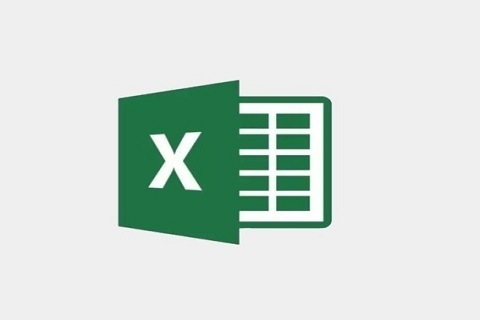excel中最常用的30个函数

1、取绝对值

=ABS(数字)

2、取整

=INT(数字)

3、四舍五入

=ROUND(数字,小数位数)

1、把公式产生的错误值显示为空

=IFERROR(A2/B2,"")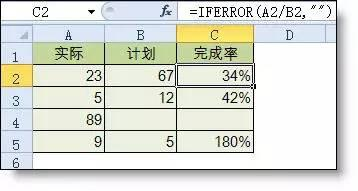2、IF多条件判断返回值

=IF(AND(A2<500,B2="未到期"),"补款","")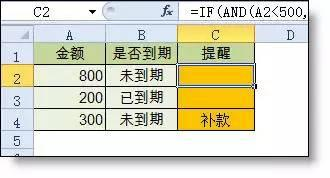1、统计两个表格重复的内容

=COUNTIF(Sheet15!A:A,A2)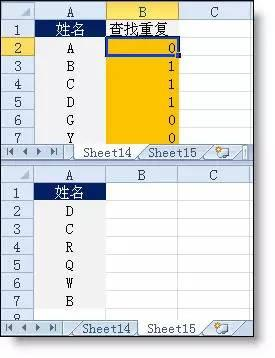2、统计不重复的总人数

=SUMPRODUCT(1/COUNTIF(A2:A8,A2:A8))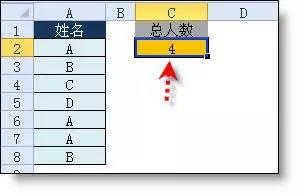1、隔列求和

=SUMIF(\$A\$2:\$G\$2,H\$2,A3:G3)

=SUMPRODUCT((MOD(COLUMN(B3:G3),2)=0)*B3:G3)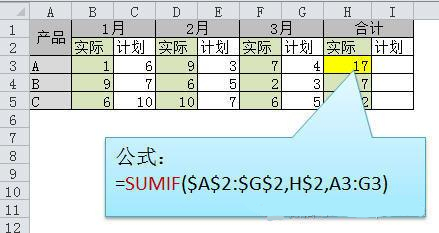2、单条件求和

=SUMIF(A:A,E2,C:C)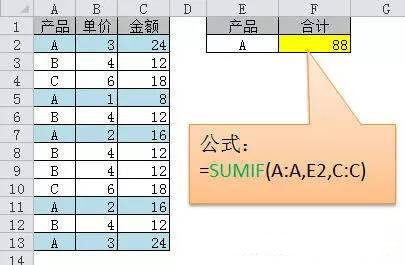3、单条件模糊求和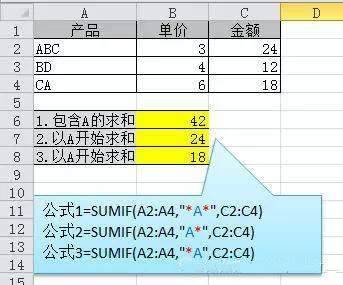4、多条件模糊求和

=SUMIFS(C2:C7,A2:A7,A11&"*",B2:B7,B11)

5、多表相同位置求和

=SUM(Sheet1:Sheet19!B2)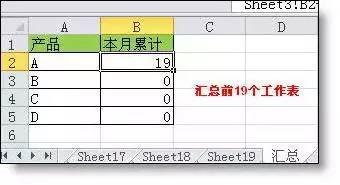6、按日期和产品求和

=SUMPRODUCT((MONTH(\$A\$2:\$A\$25)=F\$1)*(\$B\$2:\$B\$25=\$E2)*\$C\$2:\$C\$25)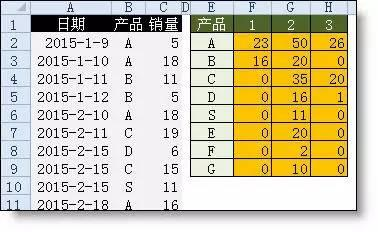1、单条件查找公式

=VLOOKUP(B11,B3:F7,4,FALSE)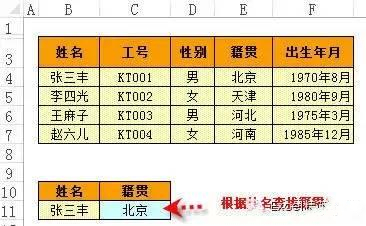2、双向查找公式

=INDEX(C3:H7,MATCH(B10,B3:B7,0),MATCH(C10,C2:H2,0))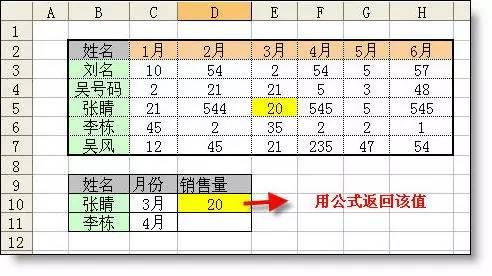3、查找最后一条符合条件的记录。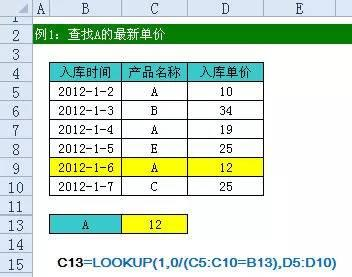4、多条件查找

5、指定区域最后一个非空值查找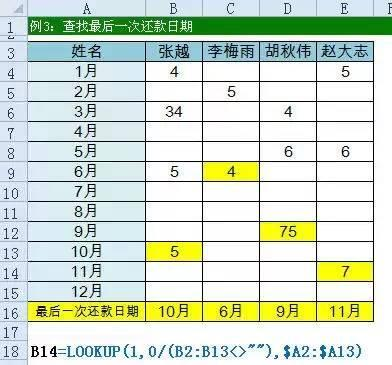6、按数字区域间取对应的值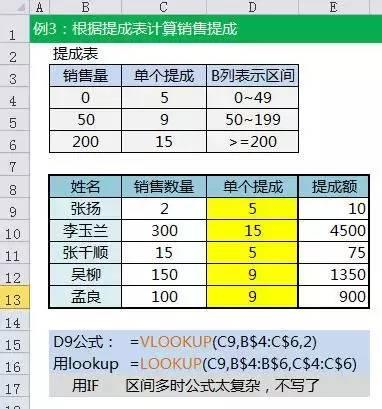1、多单元格字符串合并

=PHONETIC(A2:A7)

2、截取除后3位之外的部分

=LEFT(D1,LEN(D1)-3)

3、截取-前的部分

=Left(A1,FIND("-",A1)-1)

4、截取字符串中任一段的公式

=TRIM(MID(SUBSTITUTE(\$A1," ",REPT(" ",20)),20,20))

5、字符串查找

=IF(COUNT(FIND("河南",A2))=0,"否","是")

6、字符串查找一对多

=IF(COUNT(FIND({"辽宁","黑龙江","吉林"},A2))=0,"其他","东北")

1、两日期相隔的年、月、天数计算

A1是开始日期(2011-12-1)，B1是结束日期(2013-6-10)。计算：

datedif函数第3个参数说明：

"Y" 时间段中的整年数。

"M" 时间段中的整月数。

"D" 时间段中的天数。

"MD" 天数的差。忽略日期中的月和年。

"YM" 月数的差。忽略日期中的日和年。

"YD" 天数的差。忽略日期中的年。

2、扣除周末天数的工作日天数

=NETWORKDAYS.INTL(IF(B2

﻿